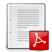# Volume 8 (2012)

Latest addition : 2 October 2012.

• ### A Two-Dimensional Relaxation Scheme for the Hybrid Modelling of Gas-Particle Two-Phase Flows

2 October 2012,

This paper presents a two-dimensional relaxation scheme for the hy- brid modelling of two-phase gas-particle flows. The method is grounded on a previous relaxation scheme that has been proposed to cope with one- dimensional flows. One of the main difficulties here is due to the fact that the Reynolds tensor occuring in the Lagrangian framework is highly anisotropic. The interface relaxation scheme mimics a previously proposed ”relaxation scheme” that involves GNL fields. The method is described and its main properties are given, including a short stability analysis, and numerical re- sults are eventually provided.

Paper presented by : Professor Thierry GallouëtA Two-Dimensional Relaxation Scheme for the Hybrid Modelling of Gas-Particle Two-Phase FlowsVoir sur HAL : https://hal.archives-ouvertes.fr/hal-01114210

• ### Rational Approximation in the sense of Kato for transport semigroups

14 May 2012,

Abstract: In this paper we mix the rational approximation procedure, which is a time approximation with approximation in the sense of Kato, which is a space approximation for linear transport equation. In 1970, H. J. Hejtmanek gave such a procedure for approximation of the linear transport equation and he proved the convergence only for explicit Euler scheme. We extend this procedure to explicit and implicit Euler, Crank-Nicolson and Predictor-Corrector schemes which have the rate 1,2 and 3 in the sense of rational approximation. Finally, we construct the numerical illustration for justifying the above rate of convergence.

Key words. Acceptable rational function. Euler’s explicit and implicit algorithms. Crank- Nicolson scheme. Predictor-Corrector algorithm. Rate of convergence. Free, absorption, production transport semigroups

Paper presented by : Professor Serge NicaiseRational Approximation in the sense of Kato for transport semigroupsVoir sur HAL : https://hal.archives-ouvertes.fr/hal-01121332

• ### A Cell-Centered Scheme For Heterogeneous Anisotropic Diffusion Problems On General Meshes

30 March 2012,

Abstract: We present a new scheme for the discretization of heterogeneous anisotropic diffusion problems on general meshes. With light assumptions, we show that the algorithm can be written as a cell-centered scheme with a small stencil and that it is convergent for discontinuous tensors. The key point of the proof consists in showing both the strong and the weak consistency of the method. The efficiency of the scheme is demonstrated through numerical tests of the 5th International Symposium on Finite Volumes for Complex Appli- cations - FVCA 5. Moreover, the comparison with classical finite volume schemes emphasizes the precision of the method. We also show the good behaviour of the algorithm for nonconforming meshes.

Key words : Heterogeneous anisotropic diffusion, general grids, finite volumes, finite elements, cell-centered scheme.

Paper presented by : Professor Thierry GallouëtVoir sur HAL : https://hal.archives-ouvertes.fr/hal-01116990